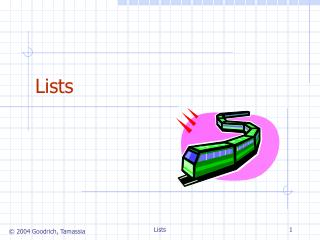DownloadDownload PresentationLists

# Lists

Download Presentation## Lists

- - - - - - - - - - - - - - - - - - - - - - - - - - - E N D - - - - - - - - - - - - - - - - - - - - - - - - - - -
##### Presentation Transcript

1. Lists Lists

2. The Position ADT models the notion of place within a data structure where a single object is stored It gives a unified view of diverse ways of storing data, such as a cell of an array a node of a linked list Just one method: object element(): returns the element stored at the position Position ADT Lists

3. The List ADT models a sequence of positions storing arbitrary objects It establishes a before/after relation between positions Generic methods: size(), isEmpty() Accessor methods (all return type Position): first(), last() prev(p), next(p) Update methods: replace(p, e) remove(p) insertBefore(p, e) insertAfter(p, e) insertFirst(e) insertLast(e) List ADT Lists

4. Vector ADTs: size() and isEmpty() elemAtRank(integer r) object replaceAtRank(integer r, object o) insertAtRank(integer r, object o) object removeAtRank(integer r) List ADT: size(), isEmpty() first(), last() prev(p), next(p) replace(p, e) remove(p) insertBefore(p, e) insertAfter(p, e) insertFirst(e) insertLast(e) Implementing Vector ADT with List ADT Lists

5. Doubly Linked List prev next • A doubly linked list provides a natural implementation of the List ADT • Nodes implement Position and store: • element • link to the previous node • link to the next node • Special trailer and header nodes elem node trailer nodes/positions header elements Lists

6. Insertion p • We visualize operation insertAfter(p, X), which returns position q A B C p q A B C X p q A B X C Lists

7. Insertion Algorithm Algorithm insertAfter(p,e): Create a new node v v.setElement(e) v.setPrev(p) {link v to its predecessor} v.setNext(p.getNext()) {link v to its successor} (p.getNext()).setPrev(v) {link p’s old successor to v} p.setNext(v) {link p to its new successor, v} return v {the position for the element e} Lists

8. p A B C D Deletion • We visualize remove(p), where p = last() A B C p D A B C Lists

9. Deletion Algorithm Algorithm remove(p): t = p.element {a temporary variable to hold the return value} (p.getPrev()).setNext(p.getNext()) {linking out p} (p.getNext()).setPrev(p.getPrev()) p.setPrev(null) {invalidating the position p} p.setNext(null) return t Lists

10. Performance • In the implementation of the List ADT by means of a doubly linked list • The space used by a list with n elements is O(n) • The space used by each position of the list is O(1) • All the operations of the List ADT run in O(1) time • Operation element() of the Position ADT runs in O(1) time Lists